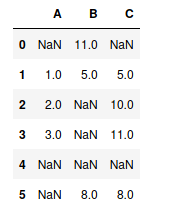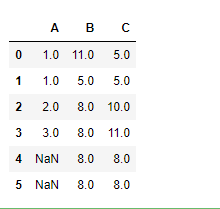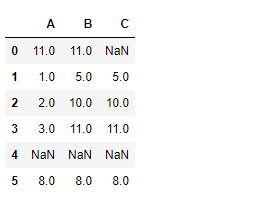Skip to content
Related Articles
Python | Pandas dataframe.bfill()
• Last Updated : 13 Feb, 2020

Python is a great language for doing data analysis, primarily because of the fantastic ecosystem of data-centric python packages. Pandas is one of those packages and makes importing and analyzing data much easier.

Pandas` dataframe.bfill()` is used to backward fill the missing values in the dataset. It will backward fill the `NaN` values that are present in the pandas dataframe.

Syntax: DataFrame.bfill(axis=None, inplace=False, limit=None, downcast=None)

Parameters:
axis : ‘rows’ or ‘columns’
inplace : boolean, default False
limit : integer value, No. of consecutive `na` cells to be populated.

Example #1: Use `bfill()` function to populate missing values `na` values in the dataframe across rows.

 `# importing pandas as pd``import` `pandas as pd`` ` `# Creating a dataframe with "na" values.`` ` `df ``=` `pd.DataFrame({``"A"``:[``None``, ``1``, ``2``, ``3``, ``None``, ``None``], ``                   ``"B"``:[``11``, ``5``, ``None``, ``None``, ``None``, ``8``],``                   ``"C"``:[``None``, ``5``, ``10``, ``11``, ``None``, ``8``]})`` ` `# Printing the dataframe``df`When `axis='rows'`, then value in current `na` cells are filled from the corresponding value in the next row. If the next row is also `na` value then it won’t be populated.

 `# Fill across the row``df.bfill(axis ``=``'rows'``)`

Output :Example #2: Use `bfill()` function to populate missing values `na` values in the dataframe across columns.

when `axis='columns'`, then the current `na` cells will be filled from the value present in the next column in the same row. If the next column is also `na` cell then it won’t be filled.

 `# importing pandas as pd``import` `pandas as pd`` ` `# Creating a dataframe with "na" values.`` ` `df ``=` `pd.DataFrame({``"A"``:[``None``, ``1``, ``2``, ``3``, ``None``, ``None``],``                   ``"B"``:[``11``, ``5``, ``None``, ``None``, ``None``, ``8``],``                   ``"C"``:[``None``, ``5``, ``10``, ``11``, ``None``, ``8``]})`` ` `# bfill values using values from next column``df.bfill(axis ``=``'columns'``)`

Output :Notice the 4th row. All values are `na` because the rightmost cell was originally `na` and there is no cell to its right from which it can populate itself. So, it could not populate the previous `na` cells as well.

Attention geek! Strengthen your foundations with the Python Programming Foundation Course and learn the basics.

To begin with, your interview preparations Enhance your Data Structures concepts with the Python DS Course. And to begin with your Machine Learning Journey, join the Machine Learning – Basic Level Course

My Personal Notes arrow_drop_up